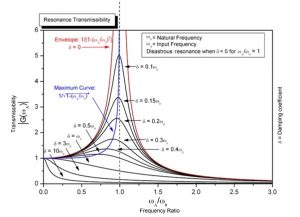# Mechanical Wave

Natural frequency of a body
Everybody in this universe has a characteristics has to tendency to vibration. When an external periodic force is applied and then amplitude and the frequency of vibration of the body depends upon its dimension and elastic property. Such as natural characteristics frequency of vibration of the body is known as natural frequency of the body. And it depends upon their shape size and elastic property.

Free vibrations
When a body capable of vibration is slightly disturbed from its equilibrium position, then this body vibration under action of restoring force. If there is no resistive force, the body keeps on vibrating forever. The amplitude of vibration of body is called free vibration. The vibration of simple pendulum in vacuum is example of free vibration.

Damped vibration
The free vibration of body is ideal concept, there always exit resistive free to reduce amplitude of vibration of the vibration body. In it energy of vibration body gradually decreases with time and finally become zero and as a result body comes to rest. Such vibration of body is known as damped vibration.

Forced vibration
In order to have sustained vibration in a body some external periodic force is to be applied. If energy supplied by the external force is equal to the energy on by the body due to damping the vibration continues i.e. the amplitude of vibration of body remains, such types is forced vibration.

Resonance
When the frequency of external source is equal to the natural frequency of the body, then body begins to vibration with maximum amplitude with the natural frequency. This kind of vibration of body is known as resonant vibration and phenomena is known as resonance, If we draw graph, frequency against amplitude, we graph’s an in figure: